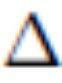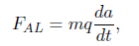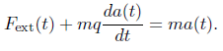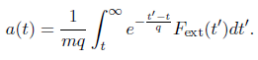# 2016PUPC普林斯顿大学物理竞赛Onsite真题与答案免费下载

### Onsite现场竞赛部分### 2016PUPC普林斯顿大学物理竞赛完整版真题免费下载

Onsite现场竞赛部分此卷共3题

Problem 1. A Jelly-Filled Universe (10 points total)

Suppose the Universe is ﬁlled with some strange gelatinous substance which produces a pseudo-drag force. A particle of mass m will experience a braking force (which we will also call the pseudo-drag force)

F = - ka

where k is some positive constant and a is the particle’s acceleration. For the following questions, assume the particle moves in one dimension for simplicity.

a) (1 point) Suppose there are no other forces on the particle. Describe the motion of the particle’s position x(t) for all times t≥0, assuming its initial speed v(0) = v0 and initial position x(0) = x0.

b) (1 point) Now suppose the particle experiences a constant external force Fext > 0 beginning at t = 0. Describe the motion of the particle’s position x(t) for all times t≥0, assuming its initial speed v(0) = v0 and initial position x(0) = x0.

c) (1 point) What is the total work W done on the particle during the time interval 0 ≤t≤T under this constant applied force? (Include the work done by the applied force and the pseudo-drag force.)

d) (2 points) Describe the physical implication of the pseudo-drag force F for the particle. In particular, describe how this pseudo-drag force di↵ers from kinetic friction (a constant braking force) and low-velocity viscous drag (a braking force proportional to the particle’s speed). Please be as speciﬁc as possible in your explanation.

e) (1 point) Suppose the particle is dropped near the Earth’s surface where there is a gravitational ﬁeld strength g. How long does it take (t) for the particle to fall through a height h? (Assume the force of gravity is mg downward, and does not depend appreciably on the height of the particle above the surface of the Earth.)

f) (2 points) It is possible to recover the same result as in part e) for the movement of the particle by removing the pseudo-drag force F and replacing g with an e↵ective gravitational ﬁeld ge↵.Find this effective field for a given value of k and m. Consider the limits in which m→0 and m→∞. Do your answers make sense? Explain.

g) (2 points) In electromagnetism, the Abraham-Lorentz force is a braking force that depends on the derivative of the particle’s acceleration:where q is some constant and m is the particle’s mass, included for convenience. Including a time-varying external force Fext(t), the equation of motion isWe can integrate this expression to ﬁnd the solution for a(t),Suppose a constant external force Fext(t)= Fext is turned on at some faraway time t = T> 0 and lasts for all t≥T . What is the acceleration a(t) of the particle for t≥0 according to the solution for a(t) given above? What is strange about this situation? Please be speciﬁc by commenting on any times of interest.

### 2016PUPC普林斯顿大学物理竞赛完整版答案免费下载